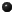# Approximations to Pi via the Dedekind eta function

F.G. Garvan
Math Department
University of Florida
Gainesville,
FL 32611

J.M. Borwein
Math Department
Simon Fraser University
Burnaby
B.C, V5A 1S6
###Mathactivated textOther available formatsRelated linksAuthor biography
Arguably the most efficient algorithm currently known for the extended precision calculation of $\pi$ is a quartic iteration due to J.M. and P.B. Borwein. In their paper, the Borwein's show how this iteration and others are intimately connected to the work of Ramanujan. This connection is shown utilizing their {\it alpha}-function which is defined in terms of theta-functions. They are able to find $p$-th order iterations based on this function using modular equations for the theta-functions. In this paper we construct an infinite family of functions $\alpha_p$. Each $\alpha_p$ gives rise to a $p$-th order iteration. For $p=4$ we obtain a quartic iteration due to the Borweins but not the one that comes from the {\it alpha}-function. For $p=3$ we obtain a cubic iteration due to the Borweins that does not come from the {\it alpha}-function. For $p=7$ we find a septic iteration that is analogous to the cubic iteration. For $p=9$ we obtain a nonic (ninth order) iteration that does not seem to come from iterating the cubic twice. Our method depends on using the computer and a symbolic algebra package to find and solve certain modular equations.